﻿ELECTROCHEMISTRY

Class 12th Electrochemistry

Exercise - 1

Q. The units of equivalent conductance are

(a) Ω cm2 equiv -1 (b) Ω-1 cm equiv -1

(c) Ω-1 cm2 equiv -1 (d) Ω-1 cm equiv

Solution : units of equivalent conductance are Ω-1 cm2 equiv -1

Hence option “C”

Q Ionisation of a weak electrolyte depends on

(a) pressure (b) volume

(c) dilution (d) none of these

Solution: with dilution dissociation of a weak electrolyte increases.

Hence option “C”

Q. Specific conductivity of a solution

(a) Increases with dilution

(b) Decreases with dilution

(c) Remains unchanged with dilution

(d) Depends on the mass of electrolyte

Solution: Specific conductivity of a solution decreases with dilution, because the number of ions per ml decrease with dilution.

Specific conductivity of a solution is the conductance of a one ml solution.

Hence option “B”

Q. The cell reaction is spontaneous, when

(a) Eored is negative (b) Eored is positive

(c) G is negative (d) G is positive

Solution: For any spontaneous process G must be negative

G = H -TS

Hence option”C”

Q. The process of Zinc plating on the iron sheet is known as

(a) Annealing (b) roasting

(c) Galvanization (d) Smelting

Solution: The process of Zinc plating on the iron sheet is known as galvanization.

Hence option “C”

Q. Pure water does not conduct electricity because it is

(a) Basic (b) almost not ionized

(c) Decomposed easily (d) Acidic

Solution: Pure water is almost unionized so does not conduct electricity

Q. If F is faraday and N is Avogadro number , then charge of electron can be expressed as

(a) F x N (b) F/N

(c) N/F (d) F2x N

Solution: one faraday is the charge on one mole of electrons.

Hence charge on one electron is F/N

Option “B”

Q. When a lead storage battery is discharged

(b) SO2 is evolved

(d) Sulphuric acid is consumed

Solution:

At Anode

Pb + H2SO4 PbSO4 + 2H+ + 2e-

At Cathode

PbO2 + H+ +SO42- +2e- PbSO4 + 2H2O

Hence Sulphuric acid is consumed when the cell is discharging.

Option “d”

Q. The laws of electrolysis were proposed by

(c) Nernst (d) Berthelot

Solution: The laws of electrolysis were proposed by faraday

Hence option “B”

Exercise - 2

Q1. In the reaction: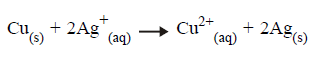The reduction half-cell reaction is

(a) Cu + 2e– Cu2+ (b) Cu – 2e– Cu 2+

(c) Ag+ + e Ag (d) Ag – e Ag+

Solution: oxidation is the loss of electron and reduction is the gain of electron

So the reduction half reaction is Ag+ + e Ag

Hence option C

Q2. When the cell reaction attains a state of equilibrium, the EMF of the cell is

(a) Zero (b) Positive (c) negative (d) not definite.

Solution: EMF of a cell is the difference in the reduction potential of the cathode and anode. When the cell attains a state of equilibrium, the difference in reduction potential of cathode and anode become zero. Hence EMF becomes zero, i.e

EMF = Ec – Ea

At equilibrium Ec = Ea

Hence EMF = Ec – Ea = 0

Hence option “A”

Q3. The metal required used to recover copper from the a solution of CuSO4 is

(a)Au (b) Fe (c) He (d) Ag

Solution: Fe can be used to recover the Copper from CuSO4 as Fe is more reactive than Cu. This is a displacement reaction and only a more reactive metal can be used to displace a less reactive metals.

Fe is higher in the activity series than Cu.

Hence option “B”

Q4. In the electrolytic cell, flow of electrons is from

(a) cathode to anode in the solution

(b) cathode to anode through external supply

(c) cathode to anode through internal supply

(d) anode to cathode through internal supply

Solution: In the electrolytic cell, oxidation (loss of electron) takes place at anode and reduction (gain of electron) takes place at cathode. Hence electrons flow from anode to cathode

Hence option “D”

(Easy) Q5. Which of the following does not conduct electricity?

(a)Fused Nacl

(b)Solid NaCl

(c)Brine solution

(d) Copper

Solution: Solid NaCl doest not conduct electricity as the ions are held tightly and are not free to move.

Q6. In the electrolysis of water, 1F of electrical energy would evolve

(a)1 mole of oxygen

(b)I gram atom of oxygen

(c)8 gram of oxygen

(d)22.4 L of oxygen

Solution: hydrolysis of water can be represented as

2H2O O2 + 4H+ + 4e-

Hence 4 Faraday of charge liberates 32 g of O2

1 F = 32/4 g = 8 grams

Hence option “C”

Q7. What is the quantity of electricity (in coulombs) required to deposit all the silver from 250 ml of 1N AgNO3 solution?

(a)2412.5

(b)24125

(c)4825

(d)48250

Solution: 250ml of 1M AgNO3 contains = 250/1000 = 0.25moles of AgNO3

Also we know that

Charge required to liberate I gram equivalent of metal = 1 F=96500c

Hence

Charge required to liberate 0.25 gram equivalent of metal =96500 x 0.25

=24125 C

Q8. The standard emf of a galvanic cell involving 2 moles of electrons in its redox reaction is 0.59V. The equilibrium constant for the redox reaction of the cell is

(a)10 20 (b) 105 (c) 10 (d) 1010

Solution: As we know Eocell = 0.0591/2 log Kc

0.59 = 0.0591/2 log Kc

Log Kc = 0.59 x 2/0.0591 = 20

Kc = 1020

Hence option “A”

Q9. The standard reduction potentials at 298K for the following half reactions are given against each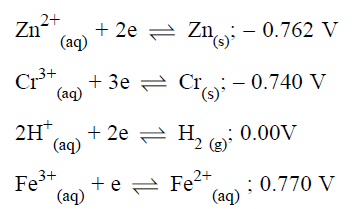Which is the strongest reducing agent?

(a) Zn(s) …….. (b) Cs(s)

(c) H2 (g) (d) Fe3+(aq)

Solution: A reducing itself gets oxidized and hence a strong reducing agent is the one that has high tendency to lose electrons and low tendency to gain the electron.

Higher reduction potential (tendency to gain the electron) means weak reducing agent.

Lower reduction potential (tendency to gain the electron) means strong reducing agent.

Among the above mentioned electrodes, Zinc has the lowest reduction potential hence strongest reducing agent.

Hence Option”A”

Q10. The quantity of electricity needed to deposit 127.08 g of copper is

(a) 1 Faraday (b) 4 Coulombs

(c) 4 Faraday (d) 1 Ampere

Solution: Cu2+ + 2e- Cu

Atomic weight of Cu is 63.5

1 mole of Cu is deposited by 2 mole of electrons i-e

63.5 gm Cu deposited by =2 F

127 gm deposited by = 4 faraday

Hence option “C”

Q11. In a solution of CuSO4 how much time will be required to precipitate 2 g copper by 0.5 ampere current?

(a) 12157.48 sec (b) 102 sec

(c) 510 sec (d) 642 sec

Solution: Cu2+ + 2e- Cu

2 faradys of electricity is required to liberate 63.5 gm of Cu

1 gram Cu = 2/ 63.5 faraday = 96500 x 2/63.5 C

Hence 2 grams = 2 x96500 x 2/63.5 C = 6078.74 C

Charge ‘Q’ ( in Coulombs) = current x time

Time = 6078.74/0.5 =12157.48 sec

Hence option “A”

Q12. The standard potentials at 25ºC for the following half reactions are given against them

Zn2+ + 2e– --- Zn, Eº = – 0.762 V

Mg2+ + 2e– -- Mg, Eº = – 2.37 V

When zinc dust is added to a solution of magnesium chloride

(a) No reaction will take place

(b) Zinc chloride is formed

(c) Zinc dissolves in the solution

(d) Magnesium is precipitated.

Solution: Here the oxidation potential of Magnesium is more than Zinc, hence Mg2+ ion can’t be displaced by the zinc. In a displacement reaction more reactive metal can displace a less reactive, reverse is not possible.

So zinc dust can’t displace magnesium from MgCl2 solution.

Hence option “A”

Q13. To a mixture containing pieces of zinc, copper and silver, 1 M H2SO4 was added. H2 gas was found to be evolved. Which of the metal/metals do you think has/have reacted?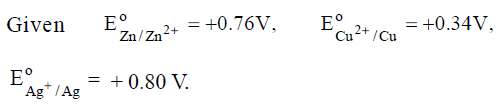(a) All the metals (b) Only Zn

(c) Both Zn and Cu (d) Only Ag.

Solution: Here standard oxidation potential of Zinc is more than hydrogen. While as standard oxidation potential of Cu and Ag is less than hydrogen.

Hydrogen has standard oxidation potential equal to zero.

Hence only Zinc can displace Hydrogen. Cu and Ag can’t.

Hence option “B”

Q14. the highest electrical conductivity of the following aqueous solutions is of

(a) 0.1 M difluoroacetic acid

(b) 0.1 M fluoroacetic acid

(b) 0.1 M chloroacetic acid

(b) 0.1 M acetic acid

Solution: since fluorine is the most electronegative atom in the whole periodic table and hence it will pull the electrons from carbon atom which in turn will pull electrons from the oxygen atom there by stabilizing the conjugate base.

More the number of F atoms, more will be the stabilization of the conjugate base.

Conjugate base of the difluoroacetic acid is the most stable and maximum ionisation. Which means the highest electrical conductivity.

Hence option A

Q15. The standard reduction potential values of the three metallic cations X, Y and Z are 0.52, – 3.03 and – 1.18V respectively. The order of reducing power of the corresponding metals is

(a) Y > Z > X ……………

(b) X > Y > Z

(c) Z > Y > X

(d) Z > X > Y

Solution: higher reduction potential of an electrode means weak reducing power. Electrodes with lower reduction potential (tendency to gain electrons) means stronger reducing agent.

A strong reducing agent is the one that has higher tendency to lose the electron or lower tendency to gain the electron.

Hence option “A”

Q16. For the cell reaction,

Cu 2+ (C1 ) + Zn (s) Zn2+ (C2 ) +Cu

of an electrochemical cell, the change in free energy, G at a given temperature is a function of

(a)ln C1

(b)ln C2/C1

(c)ln C1+C2

(d)ln C2

Solution: According to the Nernst equation

Ecell = Eocell – 0.0591/2 log [Zn2+] /[Cu2+]

G= -n F Eocell

Hence G at a given temperature is the function of ln C2/C1

Hence option “B”

Q17. For a cell reaction involving a two-electron change, the standard e.m.f. of the cell is found to be 0.295V at 25ºC. The equilibrium constant of the reaction at 25ºC will be

(a) 1 × 10–10 (b) 29.5 × 10–2

(c) 10 (d) 1 × 1010

Solution:

At equilibrium G = 0

Hence Eocell = 0.0591/n x log Keq

0.295 = 0.0591/2 x log Keq

Log Keq = 0.295 x 2/0.0591

Keq= anti log 10 = 1010

Keq = 1010

Hence option “D”

Q18. 96500 C of electricity liberates from CuSO4 solution

(a) 63.5g of Cu (b) 31.75 g of Cu

(c) 96500 g of Cu (d) 100 g of Cu

Solution: 96500 C of electricity = 1 Faraday

I faraday of electricity liberates I gram equivalent of the substance

1 gram equivalent of Cu2+ = atomic weight of Cu/ charge on ion

= 63.5/2= 31.75 g of Cu

Hence option “B”

Q19. The variation of λm (molar conductance) of acetic acid with concentration is correctly represented by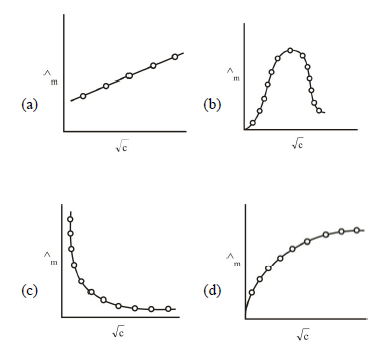Solution: For a weak electrolyte -acetic acid, with dilution dissociation of the acetic acid increases and Hence no of ions increase. So the molar conductance will increase with dilution for weak electrolytes.

Hence option “C”

Q20. Resistance of a 0.2 M solution of an electrolyte is 50 Ω. The specific conductance of the solution is 1.3 S m-1. If resistance of the 0.4 M solution of the same electrolyte is 260 Ω, its molar conductance is

(a)6250 Sm2 mol-1

(b)6.25 x 10 -4 Sm2 mol-1

(c)625 x 10-4 Sm2 mol-1

(d)62.5 Sm2 mol-1

Solution: As we know

Specific conductance = conductance x cell constant

1.3 Sm-1 = 1/50 S x cell constant

Hence cell constant = 1.3 x 50 = 65 m-1 = 65/100 cm-1

Molar conductance = 1000 x conductance x cell constant x 1/Molarity

Molar conductance = 1000 x 1/260 x 65/100 x 1/0.4 = 6.25 Scm-1 mol-1

= 6.25 x 10-4 Sm2 mol-1

Hence option “B”

Q21. The resistance of 1N solution of acetic acid is 250 Ω, when measured in a cell having a cell constant of 1.15 cm-1. The equivalent conductance (in ohm-1 cm2 equiv -1) of 1N acetic acid is

(a) 2.3

(b) 4.6

(c) 9.2

(d) 18.4

Solution: equivalent Conductance (Λeq) = k x 1000/ C

Conductivity (k) = cell constant / Resistance

= 1.15 / 250 scm-1

Hence Λeq = 1.15 x 1000/ 250 = 4.6 Ω-1 cm2 equiv -1

Hence option “B”

Q22. By diluting a weak electrolyte, specific conductivity (Kc) and equivalent conductivity (Λeq) change as

(a) Both increase

(b) Kc increases, Λeq decreases

(c) Kc decreases, Λeq increases

(d) Both decrease

Solution: When the solution of a weak electrolyte is diluted, the volume of the solution increases. Also dissociation of weak electrolyte increases with dilution and hence Λeq increases.

However, the number of current carrying ions per ml solution decreases and hence specific conductivity decreases.

Q23. The reduction potential of hydrogen half-cell will be negative if

(a) P (H2) = 1 atm and [H+] = 2.0 M

(b) P (H2) = 1 atm and [H+] = 1.0 M

(c) P (H2) = 2 atm and [H+] = 1.0 M

(d) P (H2) =2 atm and [H+] = 2.0 M

Solution: As per Nernst equation

Ered = Eored – 0.0591/n log p H2 /[H+]

Here reduction half reaction is

2 H+ + 2e- H2 hence n = 2

Eored = 0

Ered =0 – 0.0591/2 log p H2 /[H+] = 0 – 0.0591/2 log Q

For Q Ered

(a) P (H2) = 1 atm and [H+] = 2.0 M 0.25 +ve

(b) P (H2) = 1 atm and [H+] = 1.0 M 1 0

(c) P (H2) = 2 atm and [H+] = 1.0 M 2 -ve

(d) P (H2) =2 atm and [H+] = 2.0 M 0.5 +ve

Hence option “c”

Q24. E1, E2 ,E3 , are the emfs of the following three galvanic cells respectively

(i) Zn (s)Zn2+ (0.1M) ││Cu2+ (1M) │Cu (s)

(ii) Zn (s)Zn2+ (1M) ││Cu2+ (1M) │Cu (s)

(iii) Zn (s)Zn2+ (1M) ││Cu2+ (0.1M) │Cu (s)

Which one of the following is true?

(a) E2 > E1 > E3

(b) E1, > E2 > E3

(c) E3 > E1 > E2

(d) E3 > E2 > E1

Solution: As per Nernst equation, for the given cell

Ecell = Eocell – 0.0591/2 log [Zn2+] /[Cu2+]

(i)Ecell = Eocell – 0.0591/2 log [Zn2+] /[Cu2+]

= Eocell - 0.0591/2 log 1/0.1

(ii)Ecell = Eocell – 0.0591/2 log [Zn2+] /[Cu2+]

= Eocell - 0.0591/2 log 1/1

= Eocell - 0.0591/2 log 0

= Eocell

(iii)Ecell = Eocell – 0.0591/2 log [Zn2+] /[Cu2+]

= Eocell - 0.0591/2 log 0.1/1

= Eocell + 0.0591/2 log 1/0.1

= Eocell + 0.0591/2

Hence (iii) > (ii) > (i)

Q25. In the electrochemical reaction,

2 Fe 3+ + Zn Zn 2+ + 2 Fe 2+

Increasing the concentration of Fe2+

(a)Increases cell emf

(b)Increases the current flow

(c)Decreases the cell emf

(d)Ater the pH of the solution

Solution: For the cell reaction

2 Fe 3+ + Zn Zn 2+ + 2 Fe 2+

2 Fe 3+ + 2e- 2 Fe 2+

As per Nernst equation

Ecell = Eocell – 0.0591/n log [Fe2+] /[Fe3+]

Ecell = Eocell + 0.0591/n log [Fe3+] /[Fe2+]

Hence Ecell depends on the concentration of Fe2+

Increasing the concentration of Fe2+, decreases the Ecell.

Hence option”C”

Q26. Corrosion of iron is essentially an electrochemical phenomenon where the cell reactions are

(a) Fe is oxidized to Fe2+ and dissolved oxygen in water is reduced to OH-

(b) Fe is oxidized to Fe3+ and H2O is reduced to O22-

(c) Fe is oxidized to Fe2+ and H2O is reduced to O2-

(d) Fe is oxidized to Fe2+ and H2O is reduced to O2

Solution:

At anode Fe Fe2+ +2e-

At cathode O2 +2 H2O +4e- 4 OH

The overall reaction is Fe +O2+ H2O 2 Fe (OH)2

Hence option “A”

Q.27

9.65 Coulombs of electric current is passed through fused anhydrous MgCl2. The magnesium metal thus obtained is completely converted into a Grignard reagent. The number of moles of Grignard reagent obtained is

(a) 5 x10-4 (b) 1 x 10-4

(c) 5 x 10-5 (d) 1 x 10-5

Solution: Mg atomic weight 24 so 24gm of Mg = 1 mole

2 Faradys are quired to deposit 24 gm Mg

2x 96500C = 24 gm

96500 C = 12 gm

Hence 9.65 C= 12 x 9.65/ 96500 = 1.2 x 10-3 gm Mg

= 1.2 x 10-3 x 1/24 = 5 x 10-5moles of Mg

RX + Mg RMgX

5 x 10-5 5 x 10-5 moles

Hence 5 x 10-5 moles of RMgX are produced.

Option”C”

Q28. Given l/a =0.5 cm-1 ,R = 50ohm , N =1.0 .The equivalent conductance of the electrolytic cell is

(a)10 Ω-1 cm-2 g eq-1

(b)20 Ω-1 cm-2 g eq-1

(c)300 Ω-1 cm-2 g eq-1

(d)100 Ω-1 cm-2 g eq-1

Solution:

cell constant l/a= 0.5 cm-1

R = 50 ohm and N =1.0

Specific conductance (k) =1/ρ = 1/R x l/a = 0.5/ 50

Λeq = k x 1000/N = 0.5/50 x 1000/0.1

= 10 Ω-1 cm-2 g eq-1

Hence option “A”

Q29. For Strong electrolytes the plot of molar conductance Vs C is

(a)Parabolic (b) linear

(b)Sinusoidal (d) circular

Solution: For Strong electrolytes the plot of molar conductance Vs C is linear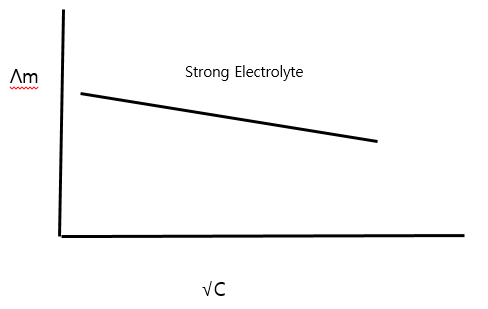Hence option “B”